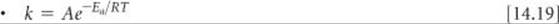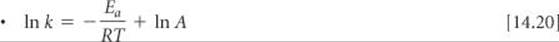﻿ ﻿CHAPTER SUMMARY AND KEY TERMS - CHEMICAL KINETICS - CHEMISTRY THE CENTRAL SCIENCE

## 14 CHEMICAL KINETICS

### CHAPTER SUMMARY AND KEY TERMS

INTRODUCTION AND SECTION 14.1 Chemical kinetics is the area of chemistry in which reaction rates are studied. Factors that affect reaction rate are the physical state of the reactants; concentration; temperature; and the presence of catalysts.

SECTION 14.2 Reaction rate are usually expressed as changes in concentration per unit time: Typically, for reactions in solution, rates are given in units of molarity per second M/s. For most reactions, a plot of molarity versus time shows that the rate slows down as the reaction proceeds. The instantaneous rate is the slope of a line drawn tangent to the concentration-versus-time curve at a specific time. Rates can be written in terms of the appearance of products or the disappearance of reactants; the stoichiometry of the reaction dictates the relationship between rates of appearance and disappearance.

SECTION 14.3 The quantitative relationship between rate and concentration is expressed by a rate law, which usually has the following form:

Rate = k[reactant 1]m[reactant 2]n...

The constant k in the rate law is called the rate constant; the exponents m, n, and so forth are called reaction orders for the reactants. The sum of the reaction orders gives the overall reaction order. Reaction orders must be determined experimentally. The units of the rate constant depend on the overall reaction order. For a reaction in which the overall reaction order is 1, k has units of s–1; for one in which the overall reaction order is 2, k has units of M–1 s–1. Spectroscopy is one technique that can be used to monitor the course of a reaction. According to Beer's law, the absorption of electromagnetic radiation by a substance at a particular wavelength is directly proportional to its concentration.

SECTION 14.4 Rate laws can be used to determine the concentrations of reactants or products at any time during a reaction. In a first-order reaction the rate is proportional to the concentration of a single reactant raised to the first power: Rate = k[A]. In such cases the integrated form of the rate law is ln[A]t = –kt + ln[A]0, where [A]t is the concentration of reactant A at time t, k is the rate constant, and [A]0 is the initial concentration of A. Thus, for a first-order reaction, a graph of ln[A] versus time yields a straight line of slope –k.

A second-order reaction is one for which the overall reaction order is 2. If a second-order rate law depends on the concentration of only one reactant, then rate = k[A]2, and the time dependence of [A] is given by the integrated form of the rate law: 1/[A]t = 1/[A]0 + kt. In this case a graph of 1/[A]t versus time yields a straight line. A zero-order reaction is one for which the overall reaction order is 0. Rate = k if the reaction is zero order.

The half-life of a reaction, t1/2, is the time required for the concentration of a reactant to drop to one-half of its original value. For a first-order reaction, the half-life depends only on the rate constant and not on the initial concentration: t1/2 = 0.693/k. The half-life of a second-order reaction depends on both the rate constant and the initial concentration of A: t1/2 = 1/k[A]0.

SECTION 14.5 The collision model, which assumes that reactions occur as a result of collisions between molecules, helps explain why the magnitudes of rate constants increase with increasing temperature. The greater the kinetic energy of the colliding molecules, the greater is the energy of collision. The minimum energy required for a reaction to occur is called the activation energy, Ea. A collision with energy Ea or greater can cause the atoms of the colliding molecules to reach the activated complex (or transition state), which is the highest energy arrangement in the pathway from reactants to products. Even if a collision is energetic enough, it may not lead to reaction; the reactants must also be correctly oriented relative to one another in order for a collision to be effective.

Because the kinetic energy of molecules depends on temperature, the rate constant of a reaction is very dependent on temperature. The relationship between k and temperature is given by the Arrhenius equation: k = AeEa/RT. The term A is called the frequency factor; it relates to the number of collisions that are favorably oriented for reaction. The Arrhenius equation is often used in logarithmic form: ln k = ln AEa/RT. Thus, a graph of ln k versus 1/T yields a straight line with slope –Ea/R.

SECTION 14.6 A reaction mechanism details the individual steps that occur in the course of a reaction. Each of these steps, called elementary reactions, has a well- defined rate law that depends on the number of molecules (the molecularity) of the step. Elementary reactions are defined as either unimolecular, bimolecular, or termolecular, depending on whether one, two, or three reactant molecules are involved, respectively. Termolecular elementary reactions are very rare. Unimolecular, bimolecular, and termolecular reactions follow rate laws that are first order overall, second order overall, and third order overall, respectively. Many reactions occur by a multistep mechanism, involving two or more elementary reactions, or steps. An intermediate is produced in one elementary step, is consumed in a later elementary step, and therefore does not appear in the overall equation for the reaction. When a mechanism has several elementary steps, the overall rate is limited by the slowest elementary step, called the rate-determining step. A fast elementary step that follows the rate-determining step will have no effect on the rate law of the reaction. A fast step that precedes the rate-determining step often creates an equilibrium that involves an intermediate. For a mechanism to be valid, the rate law predicted by the mechanism must be the same as that observed experimentally.

SECTION 14.7 A catalyst is a substance that increases the rate of a reaction without undergoing a net chemical change itself. It does so by providing a different mechanism for the reaction, one that has a lower activation energy. A homogeneous catalyst is one that is in the same phase as the reactants. A heterogeneous catalyst has a different phase from the reactants. Finely divided metals are often used as heterogeneous catalysts for solution- and gas-phase reactions. Reacting molecules can undergo binding, or adsorption, at the surface of the catalyst. The adsorption of a reactant at specific sites on the surface makes bond breaking easier, lowering the activation energy. Catalysis in living organisms is achieved by enzymes, large protein molecules that usually catalyze a very specific reaction. The specific reactant molecules involved in an enzymatic reaction are called substrates. The site of the enzyme where the catalysis occurs is called the active site. In the lock-and-key model for enzyme catalysis, substrate molecules bind very specifically to the active site of the enzyme, after which they can undergo reaction.

KEY SKILLS

• Understand the factors that affect the rate of chemical reactions. (Section 14.1)

• Determine the rate of a reaction given time and concentration. (Section 14.2)

• Relate the rate of formation of products and the rate of disappearance of reactants given the balanced chemical equation for the reaction. (Section 14.2)

• Understand the form and meaning of a rate law including the ideas of reaction order and rate constant. (Section 14.3)

• Determine the rate law and rate constant for a reaction from a series of experiments given the measured rates for various concentrations of reactants. (Section 14.3)

• Use the integrated form of a rate law to determine the concentration of a reactant at a given time. (Section 14.4)

• Explain how the activation energy affects a rate and be able to use the Arrhenius equation. (Section 14.5)

• Predict a rate law for a reaction having a multistep mechanism given the individual steps in the mechanism. (Section 14.6)

• Explain how a catalyst works. (Section 14.7)

KEY EQUATIONSRelating rates to the components of the balanced chemical equation a A + b B → c C + d DGeneral form of a rate law for the reaction A + B → productsThe integrated form of a first-order rate law for the reaction A → productsThe integrated form of the second-order rate law for the reaction A → productsRelating the half-life and rate constant for a first-order reactionThe Arrhenius equation, which expresses how the rate constant depends on temperatureLinear form of the Arrhenius equation

﻿